# What is Relative Cell Reference in Excel?

Relative reference and relative cell reference are the same things in Excel. Using it changes the cell addresses if a formula is copied in the other cells. The relative cell reference helps to repeat a formula in the columns or rows in Excel.

To give you an example of relative cell reference in Excel:

let’s say you have two values in cells A1 and B1 in a dataset. To multiply these two cells in cell C1, the formula will be: =A1*B1. Now if you want to copy down the formula in cell C2, the relative cell reference will change: =A2*B2.

## Example of Using Relative Cell Reference

Using a relative cell reference is very easy. I will explain the procedure with a simple example of a dataset. Here I have a list of food items (column A), their quantity (column B), and their price (column C). I am going to calculate the total price of the brought items.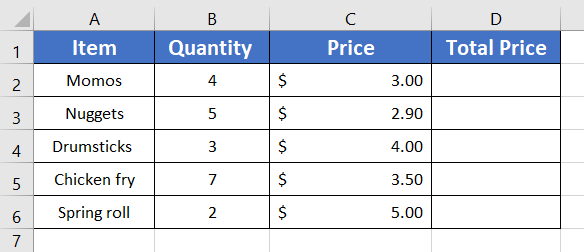Usage Guide

Step_1: Type the formula to multiply the quantities with the item prices.

Formula

`=B2*C2`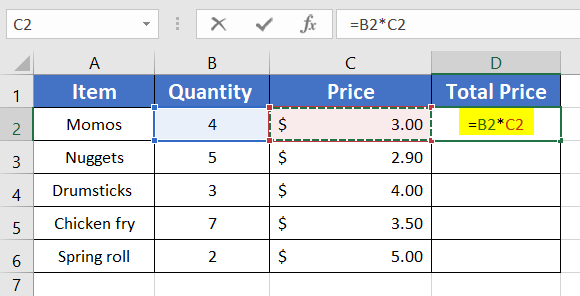Step_2: Press ENTER to apply the formula.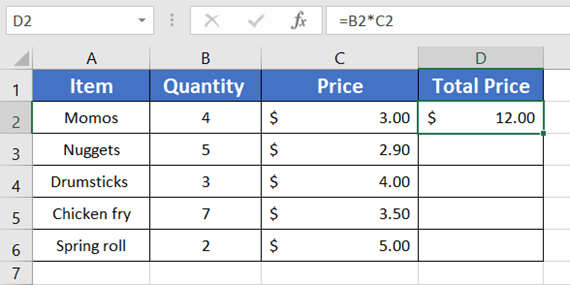Step_3: Now double-click on the Fill Handle at cell D2 to copy the formula down.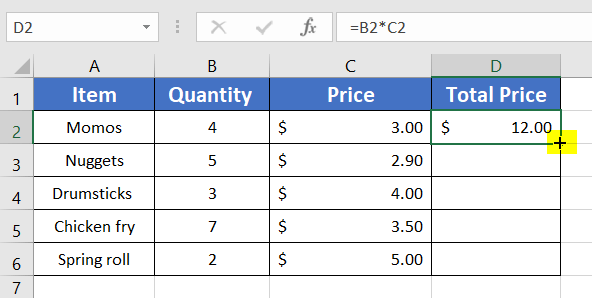You will see the formula copied in the rest of the cells of column D.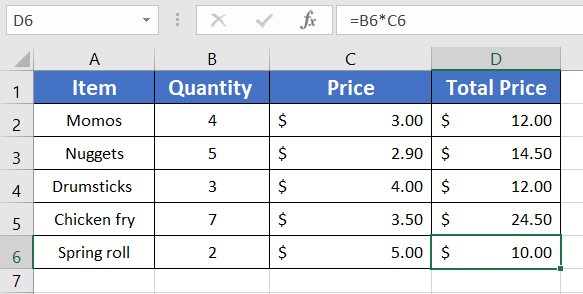Now if you want to check that all the relative cell references are correctly copied or not, follow these steps:

Step_4: Click on the Home tab.

Step_5: From the Formula Auditing group, select the Show Formula command.

You will see the formulas will become visible in the data table.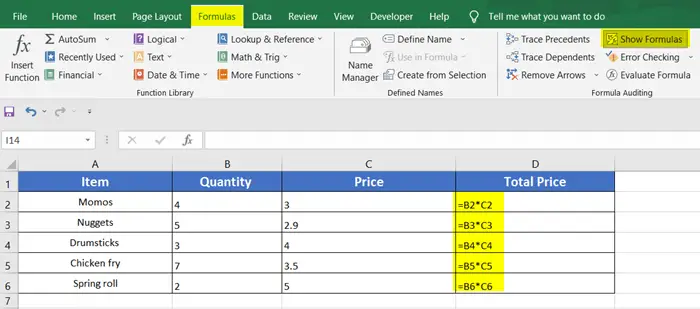## Conclusion

The relative cell reference is a very handy approach to copying formulas whether in the rows or in the columns. You just need to know the proper use of the relative reference in Excel. Check our other Blogs on our website. Thank you.